# ISEE Upper Level Math : How to find mean

## Example Questions

1 2 4 Next →

### Example Question #31 : How To Find Mean

If the mean ofconsecutive even numbers is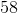, what is the smallest number of these?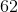Explanation:

Recall that the mean is the same as the average.

Letbe the smallest number in this set. Since these are consecutive even numbers, the other numbers can be represented as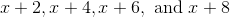.

Now, find the average of these numbers, and solve for.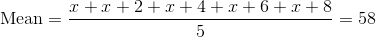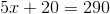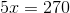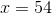The smallest number is.

### Example Question #84 : Data Analysis And Probability

A Math class took an exam.  These were their scores: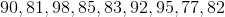Find the mean score.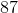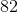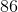Explanation:

To find the mean of a data set, we will use the following formula: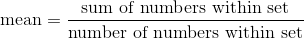Now, given the following set of Math test scoreswe can calculate the following: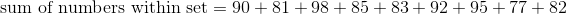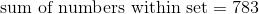Now, we will calculate the following: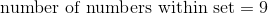because there are 9 numbers in the set.

Knowing all of this, we can substitute into the formula.  We get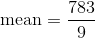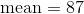Therefore, the mean score of the Math exams is 87.

### Example Question #33 : How To Find Mean

Find the mean of the following data set: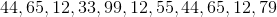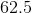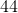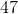Explanation:

Find the mean of the following data set:Find the mean by first summing up our terms, then dividing by the number of terms we have.

In this case, we have 11 terms, so our denominator is 11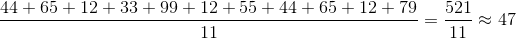So, our mean is 47

### Example Question #86 : Data Analysis

For his last six math tests, Josh scored 92, 80, 88, 94, 97, and 95. What is his mean test score?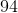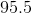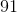Explanation:

Recall that the mean is the same as the average.

To find the average, add up all the terms that are given and divide by the number of terms there are.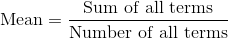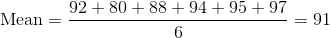His mean score is a.

### Example Question #85 : Data Analysis And Probability

In a class ofstudents, a poll was taken to see how many siblings students had. The results in the poll were then made into a table.

 Number of Siblings Number of Students with the Specific Number of Siblings 0 5 1 9 2 4 3 2

What is the mean number of siblings for this class?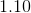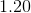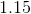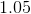Explanation:

Recall that the mean is another way of saying "average."

To find the average, we will need to find the total number of siblings the class has, then divide by the number of students in the class.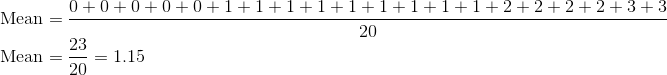Thus, the mean number of siblings the class has is### Example Question #81 : Data Analysis And Probability

Michael received the following scores on his last four French tests: 65, 58, 69, 58.

If his mean test score must be a 70 in the class to pass, what must he score on his fifth test?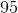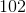Explanation:

Remember that the mean is the same as the average.

Letbe the score he needs on his fifth test. Since we already know what his average needs to be, we can set up the following equation: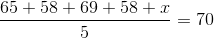Solve for.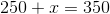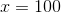Michael must scoreon his next test to pass.

### Example Question #31 : How To Find Mean

Over the course of seven games, Joanna scored the following number of points for her high school's varsity basketball team: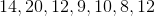In order for her to be considered for the state-wide all-star team, she needs to have a mean score of 15 points per game for the entire season. If there is only one game left in the season, how many points must Joanna score in order to make it on to the all-star team?Explanation:

Recall that the mean is just the same as the average. Letbe the number of points she must score in the last game. We can then write the following equation: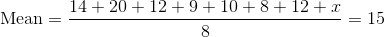Now, solve for.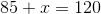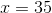Joanna must scorepoints in the next game to make the all-star team.

### Example Question #31 : Mean

Use the following data set of test scores to answer the question: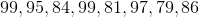Find the mean.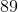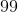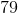Explanation:

To find the mean of a data set, we will use the following formula:Now, given the set of test scores, we can calculate the following: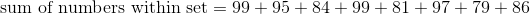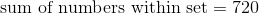Now, we will calculate the following: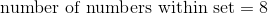because there are 8 numbers in the set.

Knowing all of this, we can substitute into the formula.  We get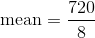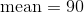Therefore, the mean score of the tests is 90.

1 2 4 Next →

### All ISEE Upper Level Math Resources